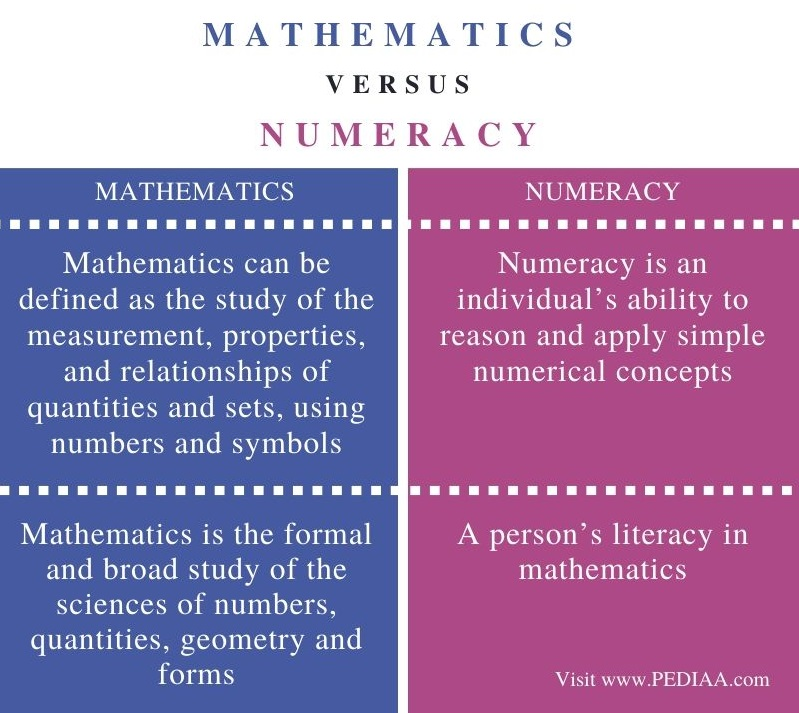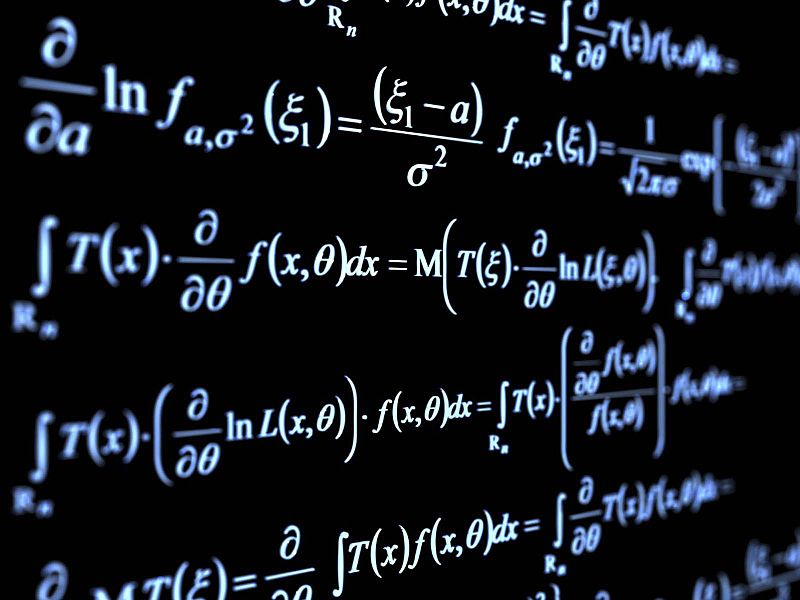# Difference Between Mathematics and Numeracy

The main difference between mathematics and numeracy is that mathematics is the broad study of numbers, quantities, geometry and forms while numeracy is one’s knowledge and skills in mathematics and its use in real life.

Mathematics can be defined as the study of the measurement, properties, and relationships of quantities and sets, using numbers and symbols (American Heritage Dictionary, 2000). Mathematics or math is not just a subject we learn at school; we need a basic knowledge of numbers, quantities, shapes and forms when dealing with real-life situations. Our ability to understand simple mathematical concepts and use them in real life is called numeracy.

### Key Areas Covered

1. What is Mathematics
– Definition, Characteristics
2. What is Numeracy
– Definition, Characteristics
3. What is the Difference Between Mathematics and Numeracy
– Comparison of Key Differences

### Key Terms

Mathematics, Numeracy## What is Mathematics

Mathematics is basically the study of numbers, patterns and shapes. It can be defined as a formal and broad study of the sciences of numbers, quantities, geometry and forms. Mathematics has developed from counting, calculation, and measurement with the use of logic and abstraction, and systematic study of shapes and motions of physical objects. Moreover, it is useful for solving real-world problems, and the knowledge of mathematics is useful for many fields such as science, business, engineering, construction, and social sciences.Furthermore, mathematics can be categorized into several major branches as below:

Arithmetic – The oldest and the most basic branch of mathematics, it deals with numbers and the basic mathematical operations like addition, subtraction, division, and multiplication.

Algebra – This is a type of arithmetic where we use unknown quantities along with numbers. We use letters of the English alphabet such as X, Y, A, B, etc. or symbols to represent unknown quantities. These symbols and letters, thus, help us to generalize formulas and find missing values algebraic equations.

Geometry: This is a practical branch of mathematics that involves shapes and sizes of objects/figures and their properties. Moreover, points, angles, lines, surfaces, and solids are the basic elements in geometry.

In addition to these branches, there are other fields in mathematics like statistics, trigonometry, applied mathematics, and analysis.

## What is Numeracy

Numeracy is basically the ability to understand and work with numbers. In other words, it’s one’s literacy in mathematics, which involves the ability to use numbers and solve problems in real life. Numeracy involves the ability to reason and understand simple mathematical concepts. It’s much more than doing sums; it involves interpreting charts, data and graphs, processing information, solving problems, understanding and explaining solutions, as well as, making decisions based on logic and reasoning.Moreover, given below are some example instances where we use numeracy:

• Making sure you have received the correct change from the grocery store
• Converting the measurements of a recipe so that you can serve an extra number of guests
• Estimating how many minutes you have to wait for the bus
• Playing board games that involve numbers
• Setting a household budget
• Measuring medicine doses, etc.

Furthermore, the opposite of numeracy is innumeracy. Innumeracy can create many problems in life since mathematical skills are an essential part of our daily lives.

## Difference Between Mathematics and Numeracy

### Definition

Mathematics can be defined as the study of the measurement, properties, and relationships of quantities and sets, using numbers and symbols, while numeracy is an individual’s ability to reason and apply simple numerical concepts.

### Basis

While mathematics is the formal and broad study of the sciences of numbers, quantities, geometry and forms, numeracy is a person’s literacy in mathematics.

### Conclusion

Mathematics or math is not just a subject we learn at school; we need a basic knowledge of numbers, quantities, shapes and forms when dealing with real-life situations. Therefore, the main difference between mathematics and numeracy is that mathematics is the broad study of numbers, quantities, geometry and forms while numeracy is one’s knowledge and skills in mathematics and its use in real life.

##### Reference:

1. “Mathematics.” Wikipedia, Wikimedia Foundation, 16 May 2020, Available here.
2. “What Is Numeracy?” National Numeracy, 18 Mar. 2020, Available here.

##### Image Courtesy:

1. “Pure-mathematics-formulæ-blackboard” By Wallpoper -(Public Domain) via Commons Wikimedia
2. “Wallet and Money Photo, Sale, Shopping, Retail, Customer, Bank” (CC0) via Wallpaper Flare### About the Author: Hasa

Hasa has a BA degree in English, French and Translation studies. She is currently reading for a Masters degree in English. Her areas of interests include literature, language, linguistics and also food.